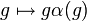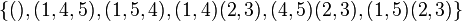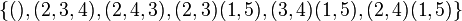# Twisted S3 in A5

This article is about a particular subgroup in a group, up to equivalence of subgroups (i.e., an isomorphism of groups that induces the corresponding isomorphism of subgroups). The subgroup is (up to isomorphism) symmetric group:S3 and the group is (up to isomorphism) alternating group:A5 (see subgroup structure of alternating group:A5).
VIEW: Group-subgroup pairs with the same subgroup part | Group-subgroup pairs with the same group part | All pages on particular subgroups in groups

## Definition

Let$G$ be the alternating group:A5, i.e., the alternating group (the group of even permutations) on the set$\{ 1,2,3,4,5 \}$.$G$ has order$5!/2 = 60$.

Consider the subgroup:$H = H_{\{1,2,3\},\{4,5 \}} = \langle (1,2,3), (1,2)(4,5) \rangle = \{ (), (1,2,3), (1,3,2), (1,2)(4,5), (2,3)(4,5), (1,3)(4,5) \}$

One way of thinking of$H$ is as follows: we first take the symmetric group on the set$\{1,2,3 \}$, which is isomorphic to symmetric group:S3, and is a subgroup of symmetric group:S5 but not of alternating group:a5. We then define a homomorphism$\alpha$ from this to the subgroup$\{ (), (4,5) \}$ of symmetric group:S5 as follows: every even permutation goes to the identity, and every odd permutation goes to$(4,5)$. We then consider the image of the group under the map$g \mapsto g\alpha(g)$. The upshot: even permutations remain as they are, and odd permutations get multiplied by$(4,5)$.

We see by construction that the map$g \mapsto g\alpha(g)$ has trivial kernel, so$H$ is isomorphic to symmetric group:S3. It is also clear that since$g$ and$\alpha(g)$ have the same sign, the product permutation is even and hence in the alternating group.$H$ has a conjugate corresponding to every possible partition of$\{ 1,2,3,4,5 \}$ into a subset of size 3 and a subset of size 2:

Subset of size 3 Subset of size 2 Corresponding conjugate of$H$
1,2,3 4,5$\! \{ (), (1,2,3), (1,3,2), (1,2)(4,5), (2,3)(4,5), (1,3)(4,5) \}$
1,2,4 3,5$\! \{ (), (1,2,4), (1,4,2), (1,2)(3,5), (2,4)(3,5), (1,4)(3,5) \}$
1,2,5 3,4$\! \{ (), (1,2,5), (1,5,2), (1,2)(3,4), (2,5)(3,4), (1,5)(3,4) \}$
1,3,4 2,5$\! \{ (), (1,3,4), (1,4,3), (1,3)(2,5), (3,4)(2,5), (1,4)(2,5) \}$
1,3,5 2,4$\! \{ (), (1,3,5), (1,5,3), (1,3)(2,4), (3,5)(2,4), (1,5)(2,4) \}$
1,4,5 2,3$\! \{ (), (1,4,5), (1,5,4), (1,4)(2,3), (4,5)(2,3), (1,5)(2,3) \}$
2,3,4 1,5$\! \{ (), (2,3,4), (2,4,3), (2,3)(1,5), (3,4)(1,5), (2,4)(1,5) \}$
2,3,5 1,4$\! \{ (), (2,3,5), (2,5,3), (2,3)(1,4), (3,5)(1,4), (2,5)(1,4) \}$
2,4,5 1,3$\! \{ (), (2,4,5), (2,5,4), (2,4)(1,3), (4,5)(1,3), (2,5)(1,3) \}$
3,4,5 1,2$\! \{ (), (3,4,5), (3,5,4), (3,4)(1,2), (4,5)(1,2), (3,5)(1,2) \}$

## Arithmetic functions

Function Value Explanation
order of whole group 60 See alternating group:A5
order of subgroup 6 See symmetric group:S3
index of the subgroup 10
size of conjugacy class 10
number of conjugacy classes in automorphism class 1

## Effect of subgroup operators

In the table below, we provide values specific to$H$.

Function Value as subgroup (descriptive) Value as subgroup (link) Value as group
normalizer the subgroup itself current page symmetric group:S3
centralizer trivial subgroup -- trivial group
normal core trivial subgroup -- trivial group
normal closure the whole group -- alternating group:A5
characteristic core trivial subgroup -- trivial group
characteristic closure the whole group -- alternating group:A5

## Conjugacy class-defining functions

Conjugacy class-defining function What it means in general Why it takes this value
Sylow normalizer for the prime$p = 3$ Normalizer of a$p$-Sylow subgroup. Since Sylow implies order-conjugate (any two$p$-Sylow subgroups are conjugate), any two normalizers thereof are also conjugate. A 3-Sylow subgroup is A3 in A5, given by$\{ (), (1,2,3), (1,3,2) \}$, and its normalizer is precisely this subgroup.

## Related subgroups

### Intermediate subgroups

There are no intermediate subgroups, because this subgroup is a maximal subgroup.

### Smaller subgroups

The values given below are specific to$H$.

Value of smaller subgroup (descriptive) Isomorphism class of smaller subgroup Number of conjugacy classes of smaller subgroup fixing subgroup and whole group Smaller subgroup in subgroup Smaller subgroup in whole group$\{ (), (1,2,3), (1,3,2) \}$ cyclic group:Z3 1 A3 in S3 A3 in A5$\{ (), (1,2)(4,5) \}$ cyclic group:Z2 3 S2 in S3 subgroup generated by double transposition in A5

## Description in terms of alternative interpretations of the whole group

Description of$G$ Corresponding description of$H$
special linear group of degree two over field:F4 Group of monomial matrices of determinant 1, i.e., matrices with determinant 1 and where each row has exactly one nonzero entry and each column has exactly one nonzero entry.
Alternatively, one of the conjugate subgroups can be thought of as a copy of$SL(2,2)$ in$SL(2,4)$, via the embedding of field:F2 in field:F4.
projective special linear group of degree two over field:F5 If we think of the field$\mathbb{F}_{25}$ as a two-dimensional vector space over field:F5, its multiplicative group embeds in$GL(2,5)$. The intersection with$SL(2,5)$ corresponds to the elements whose sixth power is the identity. Projecting down to$PSL(2,5)$ gives a cyclic group of order three, which is precisely the subgroup A3 in A5. There is an automorphism arising from the Frobenius upstairs, and introducing this as an element of$PSL(2,5)$ gives the whole group.

## Subgroup properties

### Normality-related properties

Property Meaning Satisfied? Explanation Comment
normal subgroup equals all its conjugate subgroups No (see above for other conjugate subgroups)
2-subnormal subgroup normal subgroup in its normal closure No
subnormal subgroup series from subgroup to whole group, each normal in next No
contranormal subgroup normal closure is whole group Yes
abnormal subgroup For any element$g \in G$, we have$g \in \langle H, gHg^{-1} \rangle$ Yes Follows from being a Sylow normalizer, see Sylow normalizer implies abnormal
weakly abnormal subgroup Yes Follows from being abnormal
self-normalizing subgroup equals normalizer in the whole group Yes
self-centralizing subgroup contains its centralizer in the whole group Yes
subgroup whose join with any distinct conjugate is the whole group join of the subgroup with any distinct conjugate subgroup is the whole group Yes
maximal subgroup no proper subgroup containing it Yes Note that in a finite solvable group, any maximal subgroup has prime power index (see maximal subgroup has prime power index in finite solvable group). The fact that we have a maximal subgroup here whose index is not a prime power is consistent with the fact that alternating group:A5 is not solvable.
pronormal subgroup any conjugate to it is conjugate in their join Yes
weakly pronormal subgroup Yes
paranormal subgroup Yes
polynormal subgroup Yes

### Resemblance-based properties

Property Meaning Satisfied? Explanation Comment
order-conjugate subgroup conjugate to any subgroup of the whole group of the same order Yes See subgroup structure of alternating group:A5
isomorph-conjugate subgroup conjugate to every subgroup of the whole group isomorphic to it Yes See subgroup structure of alternating group:A5
automorph-conjugate subgroup conjugate to every subgroup of the whole group automorphic to it Yes Follows from being a Sylow normalizer Sylow implies automorph-conjugate